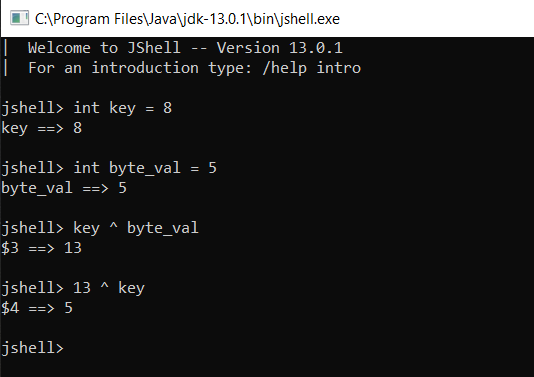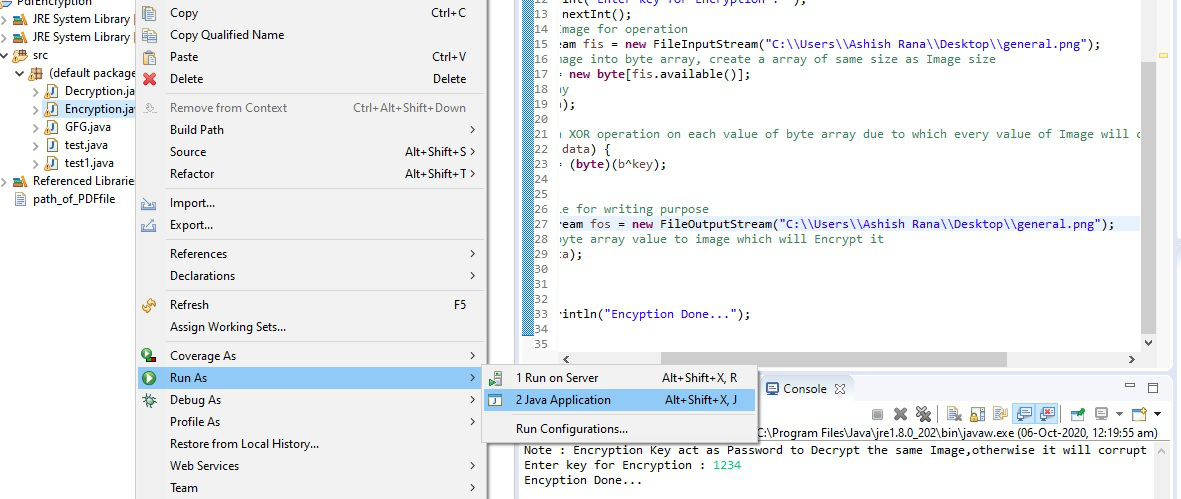Open In App

# Encrypt and Decrypt Image using Java

Encryption is the process of converting information or data into a secrete code, especially to prevent unauthorized access. In these cases also we will do the same, For encryption, we will convert the image into a byte array and after converting it we will apply XOR operation on each value of the byte array and after performing XOR operation on each and every value of byte array will be changed. After performing the operation now we will write new data in Image due to which we are unable to open the Encrypted Image. Here are key will act as a password to Encrypt and Decrypt the Image.

As we know that how to perform XOR operations now we will see how XOR operation will work here. Let’s consider an example of sample input and output.

Input:

```int key = 8
int byte_val = 5```

Operation:

```// Performing XOR operation between key and byte_val
key ^ byte_val         ```

Output:

```// Output of XOR operation
13              ```

Operation:

```// Performing XOR operation between output and key
13 ^ key                ```

Output:

```//  byte_val
5                ```

Example 1:

Here, is the screenshot of the above example. Let’s have a look.In the above example, we have clearly observed that our key = 8 and byte_val = 5, and When we perform XOR operation on key and byte_val it gives the output as 13, now if we again perform XOR operation on our output “13” and key, we get our byte_val again. The same operation performed in the case of Encryption and Decryption as well.

XOR operation performed between each and every value of byte array and key due to which all data of Image get change and due to which we are unable to open our Image. Now, whenever we apply a Decryption operation with the same key-value byte array value get the change to its original value and able to see our original Image.

Note: You can execute the given below code in any IDE offline as you need an input image location from where it can load the image as you have specified in the path for encryption and decryption.

Execute on IDE:

• Open IDE like eclipse IDE.
• Create a new project.
• Create a new class like Encryption or Decryption as required.
• Write the following code given below for encryption and Decryption in IDE.
• Just press Ctrl+S to save or you can go to file and click on save.
• Now, to run the code just select the class you want to execute like encryption then right-click.
• Run as a java application.
• Now, you will see the console window for output.

Let’s have a look at the Screenshot for the above-mentioned steps.Executable code of Encryption:

## Java

 `import` `java.io.FileInputStream;``import` `java.io.FileNotFoundException;``import` `java.io.FileOutputStream;``import` `java.io.IOException;``import` `java.util.Scanner;` `public` `class` `Encryption {``    ``public` `static` `void` `main(String[] args)``        ``throws` `FileNotFoundException, IOException``    ``{``        ``Scanner sc = ``new` `Scanner(System.in);``        ``System.out.println("Note : Encryption Key act as Password to``          ``Decrypt the same Image,otherwise it will corrupt the Image.");``     ` `        ``// Here key is act as password to Encrypt and``        ``// Decrypt the Image``        ``System.out.print(``"Enter key for Encryption : "``);``        ``int` `key = sc.nextInt();``                           ` `        ``// Selecting a Image for operation``        ``FileInputStream fis = ``new` `FileInputStream(``            ``"C:\\Users\\lenovo\\Pictures\\logo4.png"``);``                           ` `        ``// Converting Image into byte array, create a``        ``// array of same size as Image size``                           ` `        ``byte` `data[] = ``new` `byte``[fis.available()];``                           ` `        ``// Read the array``        ``fis.read(data);``        ``int` `i = ``0``;``                           ` `        ``// Performing an XOR operation on each value of``        ``// byte array due to which every value of Image``        ``// will change.``        ``for` `(``byte` `b : data) {``            ``data[i] = (``byte``)(b ^ key);``            ``i++;``        ``}``                           ` `        ``// Opening a file for writing purpose``        ``FileOutputStream fos = ``new` `FileOutputStream(``            ``"C:\\Users\\lenovo\\Pictures\\logo4.png"``);``                           ` `        ``// Writing new byte array value to image which``        ``// will Encrypt it.``                           ` `        ``fos.write(data);``                           ` `        ``// Closing file``        ``fos.close();``        ``fis.close();``        ``System.out.println(``"Encryption Done..."``);``    ``}``}`

Note: Encryption Key act as Password to Decrypt the same Image,otherwise it will corrupt the Image.

Enter key for Encryption : 1234

Encryption Done…

Decryption means the conversion of encrypted data into its original form is nothing but Decryption. In the case of Image Decryption as well we convert out encrypted Image into its original form. Here we will use XOR operation to perform decryption as well. As we observe in the above example of XOR that how we get our original value of byte array by performing XOR operation on output and key value. Same logic we will use here.

## Java

 `import` `java.io.FileInputStream;``import` `java.io.FileNotFoundException;``import` `java.io.FileOutputStream;``import` `java.io.IOException;``import` `java.util.Scanner;` `public` `class` `Decryption {``  ` `    ``public` `static` `void` `main(String[] args)``        ``throws` `FileNotFoundException, IOException``    ``{``        ``Scanner sc = ``new` `Scanner(System.in);``        ``System.out.println(``            ``"Note : Encryption Key act as Password to Decrypt the same Image,``             ``otherwise it will corrupt the Image.");``        ` `        ``System.out.print(``"Enter a key for Decryption : "``);``        ``int` `key = sc.nextInt();``          ` `        ``// Selecting a Image for Decryption.``          ` `        ``FileInputStream fis = ``new` `FileInputStream(``        ``"C:\\Users\\lenovo\\Pictures\\logo4.png"``);``        ` `        ``// Converting image into byte array,it will``        ``// Create a array of same size as image.``        ``byte` `data[] = ``new` `byte``[fis.available()];``          ` `        ``// Read the array``          ` `        ``fis.read(data);``        ``int` `i = ``0``;``          ` `        ``// Performing an XOR operation``        ``// on each value of``        ``// byte array to Decrypt it.``        ``for` `(``byte` `b : data) {``            ``data[i] = (``byte``)(b ^ key);``            ``i++;``        ``}``          ` `        ``// Opening file for writing purpose``        ``FileOutputStream fos = ``new` `FileOutputStream(``            ``"C:\\Users\\lenovo\\Pictures\\logo4.png"``);``          ` `        ``// Writing Decrypted data on Image``        ``fos.write(data);``        ``fos.close();``        ``fis.close();``        ``System.out.println(``"Decryption Done..."``);``    ``}``}`

Note: Encryption Key act as Password to Decrypt the same Image,otherwise it will corrupt the Image.

Enter a key for Decryption : 1234

Decryption Done…

By using the same logic of Encryption and Decryption of Image we can also create a small GUI project as well. Let’s have a look.